# Civil Engineering - RCC Structures Design

### Exercise :: RCC Structures Design - Section 1

11.

If W is the load on a circular slab of radius R, the maximum circumferential moment at the centre of the slab, is

 A.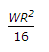B.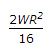C.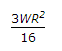D. zero E. none of these.

Explanation:

No answer description available for this question. Let us discuss.

12.

If a bent tendon is required to balance a concentrated load W at the centre of the span L, the central dip h must be at least

 A.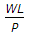B.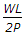C.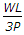D.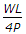E.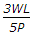Explanation:

No answer description available for this question. Let us discuss.

13.

For M 150 mix concrete, according to I.S. specifications, local bond stress, is

 A. 5 kg/cm2 B. 10 kg/cm2 C. 15 kg/cm2 D. 20 kg/cm2 E. 25 kg/cm2

Explanation:

No answer description available for this question. Let us discuss.

14.

If the average bending stress is 6 kg/cm2 for M 150 grade concrete, the length of embedment of a bar of diameter d according to I.S. 456 specifications, is

 A. 28 d B. 38 d C. 48 d D. 58 d E. 95 d

Explanation:

No answer description available for this question. Let us discuss.

15.

Bottom bars under the columns are extended into the interior of the footing slab to a distance greater than

 A. 42 diameters from the centre of the column B. 42 diameters from the inner edge of the column C. 42 diameters from the outer edge of the column D. 24 diameter from the centre of the column